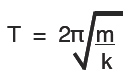Simple Harmonic Motion (SHM) for a spring

The SHM of a mass oscillating on a spring is the most common example used in schools and colleges because it is simple and easy to set up and it completely matches the conditions for simple harmonic motion.  That is, providing the mass of the spring is insignificant compared to the mass on the end.

The equation for the oscillation of a spring, is:The spring constant

is the force required to displace the mass (stretch the spring) by one metre.  We can use this equation to calculate the time of one oscillation of a mass on the spring if we know the spring constant K. The value of k is the force needed to stretch the spring by 1m, an amount which would almost certainly destroy the spring.

Measuring the extension of a spring

We actually find the value by measuring the force for a smaller extension and calculating the value for 1 metre. To do this we graph the results and so avoid any major errors. The points plotted should all be very close to or on a straight line.

The constant is then  k (N/m) = force /extension

The time period

of a spring is for one complete oscillation. From a point and back to that same point traveling in the same direction. For example from the centre to the bottom, back to the centre, to the top and back to the centre again. So that we get an accurate value of one oscillation we measure a lot (perhaps ten or twenty) and then divide. The end points of the oscillation are accurately marked by a fiducial point.